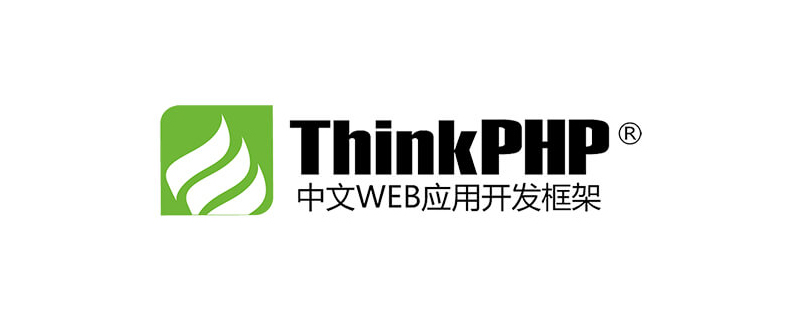【亿码酷站-编程开发教程】收集全网优质教程及源码资源！ThinkPHP`Container` 类提供了静态方法 `get()` ，可以根据类名或类的别名获取实例，会保持创建完成的实例，避免重复创建。下面实现这个方法，修改 `Container.php` ，添加以下代码。

```//     * ThinkPHP 5 与 6 在此处参数一致//     * @param string \$abstract//     * @param array \$vars//     * @param bool \$newInstance//     */
public static function get(string \$abstract, array \$vars = [], bool \$newInstance = false)
{
return static::getInstance()->make(\$abstract, \$vars, \$newInstance);
}```

```protected static \$instance;public static function getInstance()
{
// 创建自身实例
if (is_null(static::\$instance)) {
static::\$instance = new static;
}
return static::\$instance;
}```

```public function make (string \$abstract, array \$vars = [], bool \$newInstance = false)
{
// 这里的 \$abstract 是包含有命名空间的类名
if (isset(\$this->bind[\$abstract])) {
\$abstract = \$this->bind[\$abstract];
}

// 如果已经实例化直接返回
if (isset(\$this->instances[\$abstract]) && !\$newInstance) {
return \$this->instances[\$abstract];
}

// 如果就创建
\$object = \$this->invokeClass(\$abstract, \$vars);

// 保存实例
if (!\$newInstance) {
\$this->instances[\$abstract] = \$object;
}

return \$object;
}```

```    protected \$bind = [
'app' => App::class,
'config' => Config::class,
'request' => Request::class
];```

```public function invokeClass (string \$class, array \$vars = [])
{
// \$vars 为构造函数的参数
return new \$class();
}```

`require __DIR__ . '/../core/base.php';use think\Request;\$req = \think\Container::get('request');var_dump(\$req instanceof Request);`

```    public function __get(\$abstract)
{
// 返回容器的类实例
return \$this->make(\$abstract);
}public function __set(\$abstract, \$instance)
{
if (isset(\$this->bind[\$abstract])) {
\$abstract = \$this->bind[\$abstract];
}
// 装入容器
\$this->instances[\$abstract] = \$instance;
}```

`index.php` 进行测试

`\$container = \think\Container::getInstance();// 获取容器中的实例，输出对象var_dump(\$container->request);// 装入容器\$container->contianerName = \$container;var_dump(\$container->contianerName);`